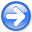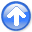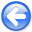Fast log-decon with a quasi-Newton solver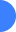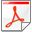Next: A fair warning Up: Two solvers Previous: The slow steepest-descent method

## The fast L-BFGS method

The L-BFGS method is a member of the quasi-Newton family: it updates at each iteration an approximation of the inverse Hessian. This technique is very cost effective: given the most recent history of gradient and model vectors (usually around 5) kept in memory, the quasi-Newton search direction(inverse Hessian times the gradient) is computed directly with simple vector multiplications. Therefore, the L-BFGS solver can be used for large non-linear problems (Nocedal, 1980).

Contrary to steepest descent where the step length is estimated with a Newton-search technique, the step length in L-BFGS is computed such that sufficient decrease of the error and of the local curvature is attained (so called ``Wolfe conditions''). The appendix shows the L-BFGS solver in more details. The L-BFGS code can be downloaded at http://users.eecs.northwestern.edu/~nocedal/lbfgs.html The pseudo-code below shows both the steepest-descent and L-BFGS algorithms.

```U = 0.        # or other initializations
Remove the mean from U(omega).

Iteration {
dU = 0
Compute dU
Remove mean from dU
du = FT(dU)
if (steepest descent)
{
Compute alfa with Newton iterations
u  = u + alfa*du
}
else if (L-BFGS)
{
Compute alfa with More and Thuente method
u  = u + alfa*Qdu # Q = inverse approximate Hessian
}
}
```

2012-10-29